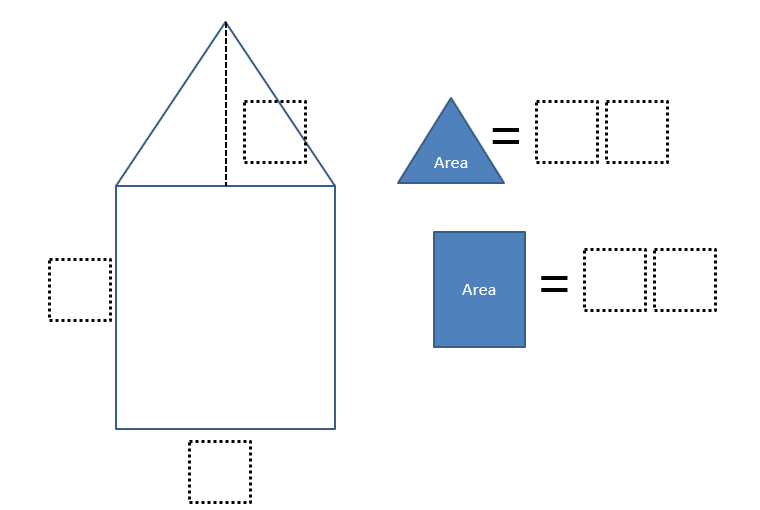# Area of a House

Directions: Using the digits 0 – 9, at most one time each, fill in the blanks to make a true statement. (Note: Image is not to scale)### Hint

Which shapes two shapes can this house be decomposed into?
Which formulas can be helpful in determining areas of these shapes?

### Answer

Answer 1: The base of the rectangle is 8, and the height is 7. The area is 56. The height of the triangle is 3. The area is 12.
Answer 2: The base of the rectange is 8, and the height is 9. The area is 72. The height of the triangle is 4. The area is 16.
Answer 3: The base of the rectangle is 8, and the height is 7. The area is 56. The height of the triangle of 1. The area is 04.

Source: Owen Kaplinsky

## Ordering Rational Numbers

Directions: Using the digits 1 to 9 at most once each, fill in the boxes …